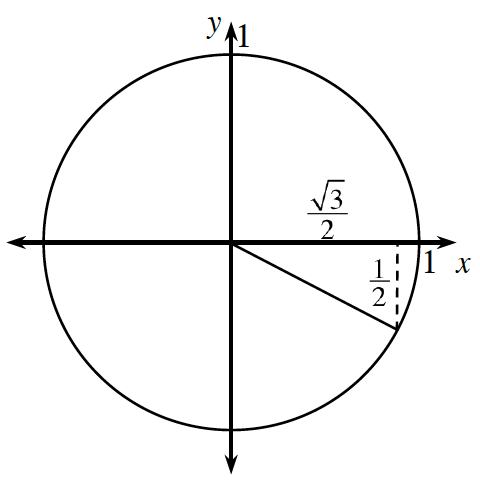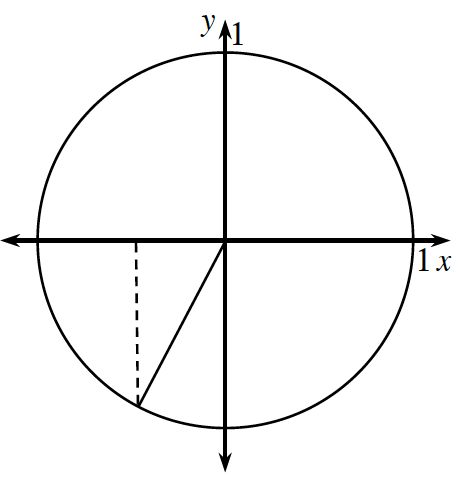### Home > PC3 > Chapter 6 > Lesson 6.1.1 > Problem6-12

6-12.

Determine the exact values of the following trigonometric expressions.

1. $\cos\left(\frac{3\pi}{4}\right)$

Sketch a unit circle with a $45^\circ-45^\circ-90^\circ$ triangle in Quadrant II.

1. $\sin\left(\frac{11\pi}{6}\right)$1. $\sin\left(-\frac{2\pi}{3}\right)$1. $\tan\left(\frac{5\pi}{4}\right)$

Sketch a unit circle with a $45^\circ-45^\circ-90^\circ$ triangle in Quadrant III.